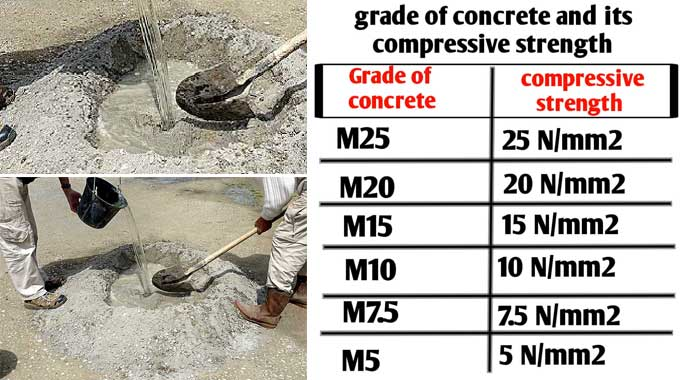# How to add the Right Quantity of Water in M20 Grade ConcreteA concrete mix consists of cement, sand, aggregates, and water. In order to achieve desired strength in concrete, the Water-Cement ratio plays a great role. The proportion of water and cement varies by grade. The quantity of water required for each grade of concrete mix design varies. Concrete may shrink and creep if excessive water is added.

Make sure that the M20 concrete has the correct amount of water. Unless you do this, you will have to repeat the entire process.

Several factors need to be taken into consideration before you make concrete in the construction field. Concrete is made by mixing cement sand aggregate, water, and some admixtures in the required proportions to achieve the desired strength; this is what makes the workable paste with the required consistency for pouring.

### Strength & Workability of Concrete

A concrete mix's strength and workability are determined by its water-cement ratio, which falls between 0.4 and 0.6 for nominal mixes, as the water-cement ratio is defined as the weight ratio of water to cement, also known as the water cement ratio.

In general, water requirements are stated for a particular mix. Depending on the water-to-cement ratio, concrete can be both stronger and more workable or more durable.

A cement concrete cube of size 15cm x 15cm x 15cm is composed of cement, sand, and aggregates and the cube are examined after 28 days of curing to determine that it has a compressive strength of 20 MPA or 20 N/mm2. M20 concrete was designed with a nominal mix ratio of 1:1.5:3.

### Quantity of Water Needed in m20 Grade Concrete

Cement, sand, and gravel, which are the three most important ingredients in concrete, have almost the same density, so they are mixed 1:1.5:3. To get 20 MPA strength, mix one-part cement to 1.5 parts sand and three parts aggregate. Under mild exposure conditions, cement should be watered up to 55 percent of its weight with water.

Water Calculation for m20 Concrete

Assume the wet quantity of a nominal mix of M20 concrete is about 1 m3. So the dry volume will equal 1 x 1.54 = 1.54 m3.

To determine the amount of cement in a mix, multiply the total proportion by 1/5.5 of the dry volume, 1 + 1.5 + 3 = 5.5.

The water required for 1 m3 of the nominal mix of m20 concrete is about 240 liters, according to math calculations such as 403 × 0.55 = 220 liters.

The water concrete ratio is about 0.55 for a normal mix of M20 concrete, so the amount of water needed for a 50kg bag of cement is about 28 liters. The amount of water in an m20 concrete mix equals = (220 ÷ 403) × 50 = 28 liters.

### Maximum Amount of Water

A nominal mix of M20 grade concrete requiring a 50kg bag of cement requires about 30 liters of water under mild exposure conditions. The maximum water-cement ratio is 0.60, so 240 liters of water are required for 403 kg cement, so = (240÷403) × 50 = 30 liters.

To learn more, watch the following video tutorial.

Video Source: Civil Engineers

### Minimum Amount of Water

In extreme exposure conditions, a nominal mix of M20 grade concrete requires about 20 liters of water per 50kg bag of cement. A water-cement ratio of 0.40 means that 403kg cement requires 160 liters of water, so water is required for 50kg cement equal to (160÷403) × 50 = 20 liters.